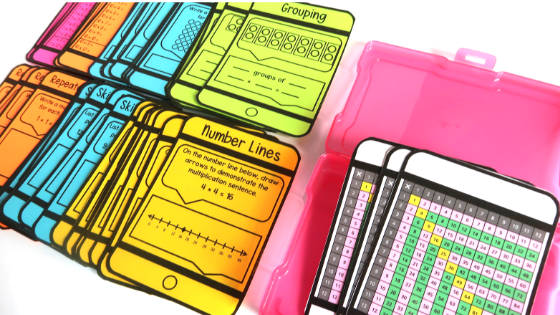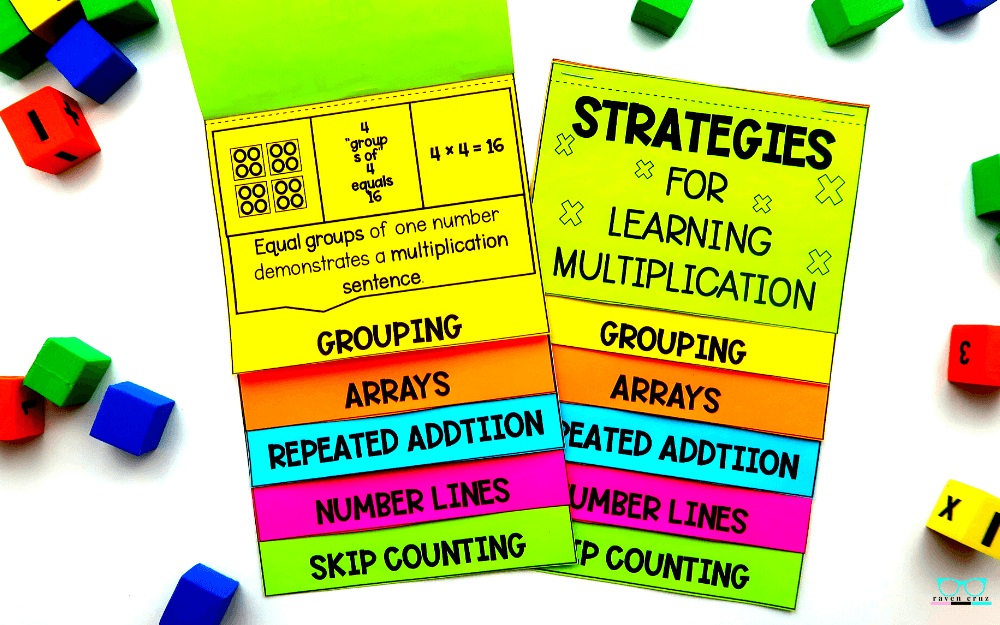5 Strategies for Teaching Multiplication With Ease - Raven Cruz

# 5 Strategies for Teaching Multiplication With Ease

There are 5 multiplication strategies I use for teaching multiplication with ease. These multiplication strategies include; grouping, arrays, repeated addition, skip counting, or number lines

Read more about the multiplication strategies below. Also, see my resource that compliments theses strategies.

## Multiplication by Grouping

The first multiplication strategy is learning multiplication by grouping is the process of separating objects into equal groups. Most commonly when using equal groups, repeated addition is also used. This method is one of the most straightforward ways for students to comprehend the strategy of multiplication by grouping.

As you can see in the example below there are 3 equal groups of 2 cookies. Students can use repeated addition to solve a multiplication problem like the one below.

In the classroom teachers can use manipulatives such as; erasers, counters, small blocks, or even drawing on dry erase boards to practice the strategy of grouping and repeated addition.2 + 2 + 2 = 6

## Multiplication by Arrays

Second, are arrays. Arrays are an arrangement of objects arranged in rows and columns.

The arrangement of cookies shown below shows the systematic way to introduce arrays.  The student will first learn a 1 × 4 array, 2 × 4 array, 3 × 4 array, 4 × 4 array, and so on... A larger arrangement of objects can also be broken down into arrays to preform many different types of multiplication, such as properties of multiplication.

Also, making arrays in the classroom can be fun AND engaging for students. Using colorful objects, such as; erasers, counters, and blocks to build arrays can keep all types of learners engaged and ready to learn.1 × 42 × 43 × 44 × 4

Third, is repeated addition. We touched on using repeated addition with grouping above when we grouped 2 cookies in groups of three. Both learning using grouping and learning using arrays are complimentary to each other.

3 + 3 + 3 + 3 + 3 + 3 = 18

## Multiplication by Skip Counting

Last, learning multiplication by number lines uses the next strategy - skip counting. Skip counting is not just for providing students a way to count faster, it also helps them solve multiplication problems.

Starting at 0 students count in intervals until reaching the desired number. Students can also point out the pattern being used when skip counting.

0, 5, 10, 15, 20, 25, 30...

## Multiplication by Number Lines

Fourth, is learning multiplication by number lines. Students will take "jumps" on the number line. These jumps help students solve  multiplication problems by skip counting.

Number lines are an exceptional way to teach number sense as well.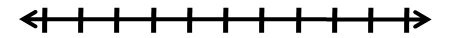2  4  6  8 10.....

## Resources to Teach Multiplication

In conclusion, the 5 strategies to teach multiplication with ease include grouping, arrays, repeated addition, skip counting, and number lines.

I offer several resources in my store that help teach these strategies. Browse the photographs below - each photo is hyper-linked to that resource. You can also buy them bundled in Multiplication Strategies Bundle and save \$4.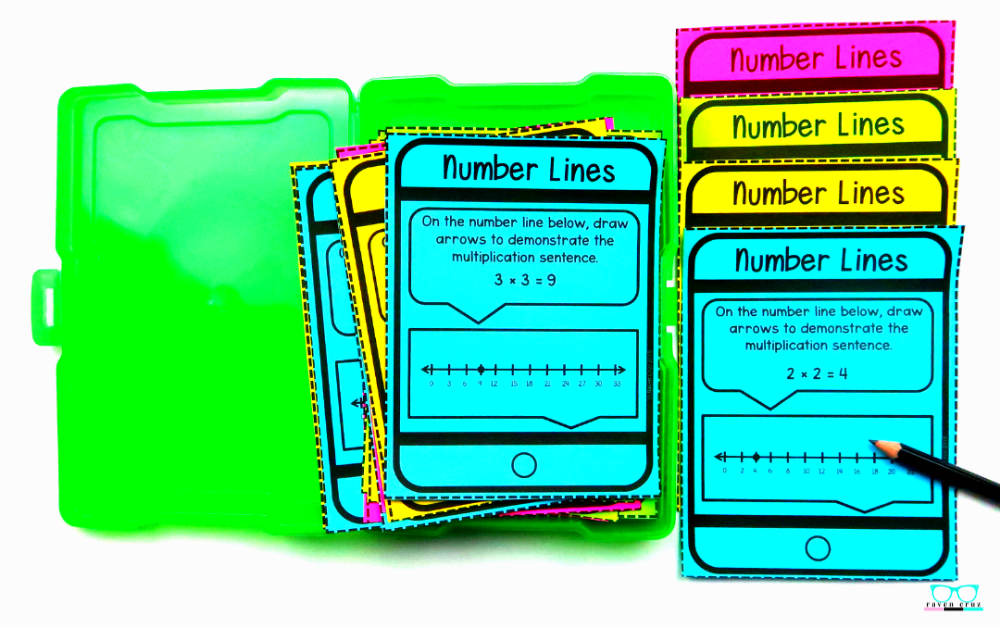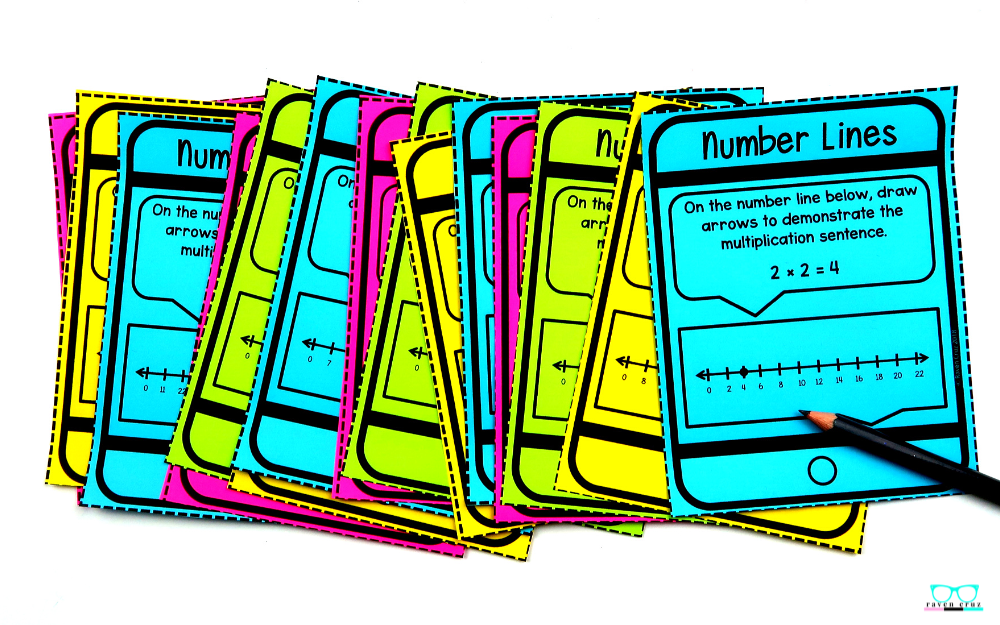## Buy the BUNDLE and SAVE!